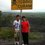# $\sqrt{i}$

Why is $\sqrt{i} = \frac{\sqrt{2}}{2} + \frac{\sqrt{2}}{2}i$6 years, 9 months ago

This discussion board is a place to discuss our Daily Challenges and the math and science related to those challenges. Explanations are more than just a solution — they should explain the steps and thinking strategies that you used to obtain the solution. Comments should further the discussion of math and science.

When posting on Brilliant:

• Use the emojis to react to an explanation, whether you're congratulating a job well done , or just really confused .
• Ask specific questions about the challenge or the steps in somebody's explanation. Well-posed questions can add a lot to the discussion, but posting "I don't understand!" doesn't help anyone.
• Try to contribute something new to the discussion, whether it is an extension, generalization or other idea related to the challenge.

MarkdownAppears as
*italics* or _italics_ italics
**bold** or __bold__ bold
- bulleted- list
• bulleted
• list
1. numbered2. list
1. numbered
2. list
Note: you must add a full line of space before and after lists for them to show up correctly
paragraph 1paragraph 2

paragraph 1

paragraph 2

[example link](https://brilliant.org)example link
> This is a quote
This is a quote
    # I indented these lines
# 4 spaces, and now they show
# up as a code block.

print "hello world"
# I indented these lines
# 4 spaces, and now they show
# up as a code block.

print "hello world"
MathAppears as
Remember to wrap math in $$ ... $$ or $ ... $ to ensure proper formatting.
2 \times 3 $2 \times 3$
2^{34} $2^{34}$
a_{i-1} $a_{i-1}$
\frac{2}{3} $\frac{2}{3}$
\sqrt{2} $\sqrt{2}$
\sum_{i=1}^3 $\sum_{i=1}^3$
\sin \theta $\sin \theta$
\boxed{123} $\boxed{123}$

Sort by:

$\displaystyle i=e^{i\pi/2} \Rightarrow \sqrt{i}=e^{i\pi/4}=\cos(\pi/4)+i\sin(\pi/4)=\frac{\sqrt{2}}{2}+\frac{\sqrt{2}}{2}i$

- 6 years, 9 months ago

remember taking root will give both positive and negative solutions

- 6 years, 9 months ago

I completely agree though you forgot to put in the $i$ until the end: $i=e^{\pi i/2}$ Voted up. :D

- 6 years, 9 months ago

Thanks! :)

- 6 years, 9 months ago

Actually, $i$ has two square roots, just as any real number does. These are the two roots of $z^2=e^{\pi i/2}$. One of the roots is $e^{\pi i/4}=\frac{\sqrt{2}}{2}+\frac{\sqrt{2}}{2}i$ as noted previously. The other is $e^{5\pi i/4}=\frac{\sqrt{2}}{2}-\frac{\sqrt{2}}{2}i$. Doubling the argument of both of these complex numbers and squaring the modulus indeed gives $e^{\pi i/2}$.

- 6 years, 9 months ago

There is some method with which we agree on which root we're talking about with radicals, making radicals ambiguous but fractional exponents not so.

Sadly, I don't know exactly how we do decide. Could anyone enlighten me?

- 6 years, 9 months ago

The "principal root" of x describes the positive root, so if you're given $\sqrt{4}$ then it is asking for the positive answer which is $2$ and not $-2$. but when dealing with complex numbers it gets.. well, more complex. Neither root is more "important" than the other, so you don't tend to worry about it as much.

- 6 years, 9 months ago

and for higher roots? (e.g. $\sqrt{2}$)

- 6 years, 9 months ago

You're missing a minus sign:

$e^{5 \pi i / 4} = -\frac{\sqrt{2}}{2} - \frac{\sqrt{2}}{2}i$

- 6 years, 9 months ago

let $\sqrt{i}$ = $x$

we have $x^{2}$ = $i$

converting i to polar form, we have

$x^{2}$ = $\cos \frac{\pi}{2} + i\sin \frac{\pi}{2}$

using Demoivre's Theorem

we have $x_{1} = \cos \frac{\pi}{4} + i\sin \frac{\pi}{4} = \frac{\sqrt2}{2} + \frac{\sqrt2}{2} i$ and $x_{2} = \cos \frac{5\pi}{4} + i\sin \frac{5\pi}{4} = -\frac{\sqrt2}{2} -\frac{\sqrt2}{2} i$

- 6 years, 9 months ago

$\sqrt{i}$ is a complex number, so it can be written in the form $a+bi$ where $a$ and $b$ are real numbers.

Squaring gives $(a^2-b^2)+2abi$. It follows that $2ab=1$ and $a^2=b^2$.

From the second we have that $a=b$ or $a=-b$.

Substituting in the first, we get $a^2=\frac{1}{2}$ or $a^2=-\frac{1}{2}$

So $a=\pm \frac{1}{2} \sqrt{2}$ or $a=\pm \frac{1}{2} \sqrt{2}*i$.

and $b=\pm \frac{1}{2} \sqrt{2}$ or $b=\mp \frac{1}{2} \sqrt{2}*i$.

Using these in our original expression $a+bi$, we get only two distinct solutions:

$\frac{1}{2} \sqrt{2} + \frac{1}{2} \sqrt{2}*i$ and $- \frac{1}{2} \sqrt{2} - \frac{1}{2} \sqrt{2}*i$.

I think usually the first is chosen as THE root of $i$, but only because it's closest to $(1,0)$.

- 6 years, 9 months ago